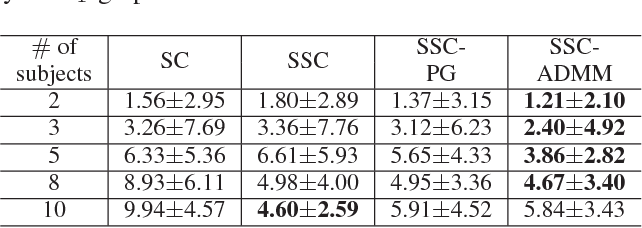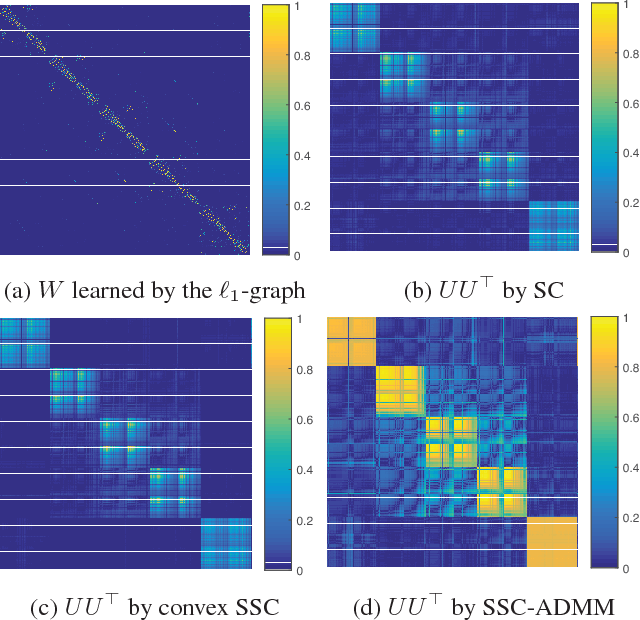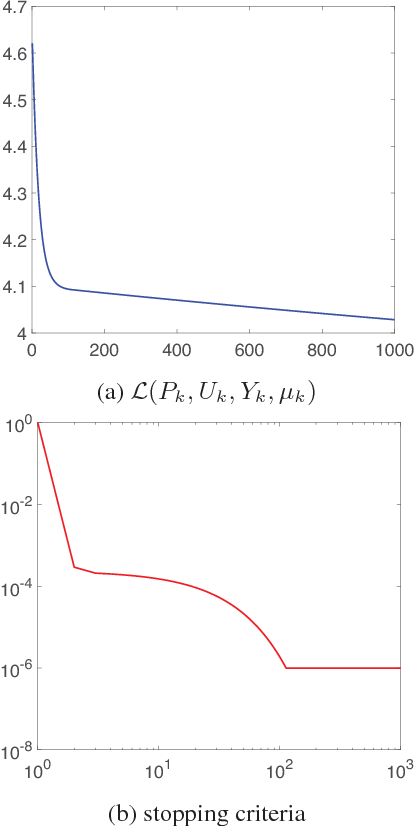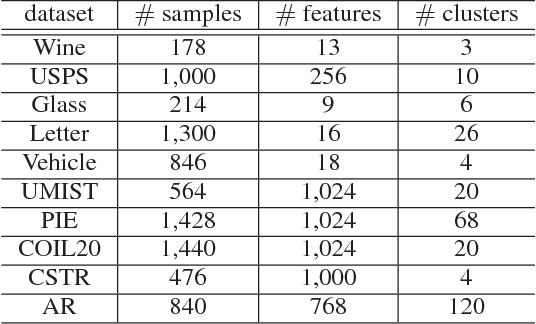Spectral Clustering (SC) is a widely used data clustering method which first learns a low-dimensional embedding $U$ of data by computing the eigenvectors of the normalized Laplacian matrix, and then performs k-means on $U^\top$ to get the final clustering result. The Sparse Spectral Clustering (SSC) method extends SC with a sparse regularization on $UU^\top$ by using the block diagonal structure prior of $UU^\top$ in the ideal case. However, encouraging $UU^\top$ to be sparse leads to a heavily nonconvex problem which is challenging to solve and the work (Lu, Yan, and Lin 2016) proposes a convex relaxation in the pursuit of this aim indirectly. However, the convex relaxation generally leads to a loose approximation and the quality of the solution is not clear. This work instead considers to solve the nonconvex formulation of SSC which directly encourages $UU^\top$ to be sparse. We propose an efficient Alternating Direction Method of Multipliers (ADMM) to solve the nonconvex SSC and provide the convergence guarantee. In particular, we prove that the sequences generated by ADMM always exist a limit point and any limit point is a stationary point. Our analysis does not impose any assumptions on the iterates and thus is practical. Our proposed ADMM for nonconvex problems allows the stepsize to be increasing but upper bounded, and this makes it very efficient in practice. Experimental analysis on several real data sets verifies the effectiveness of our method.

* Proceedings of the AAAI Conference on Artificial Intelligence (AAAI). 2018
Click to Read Paper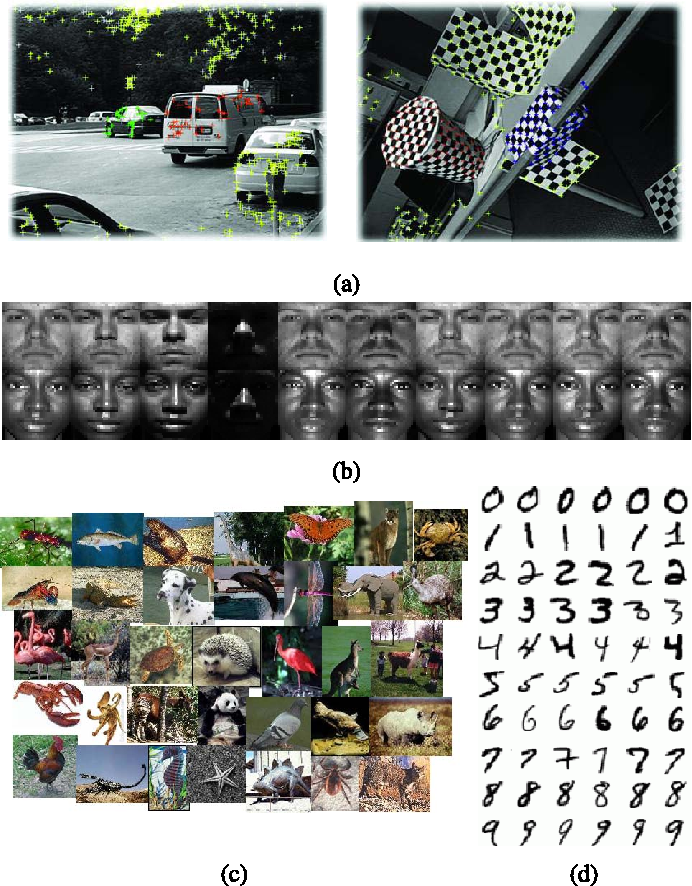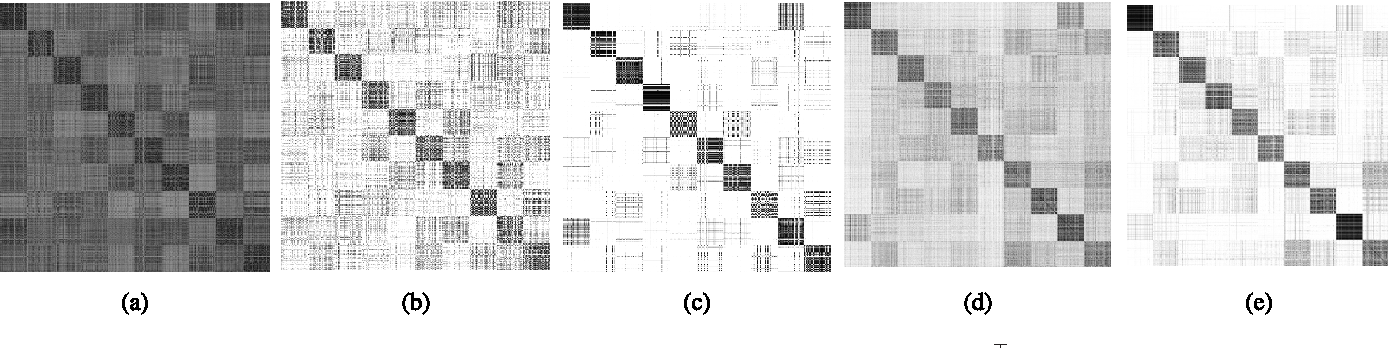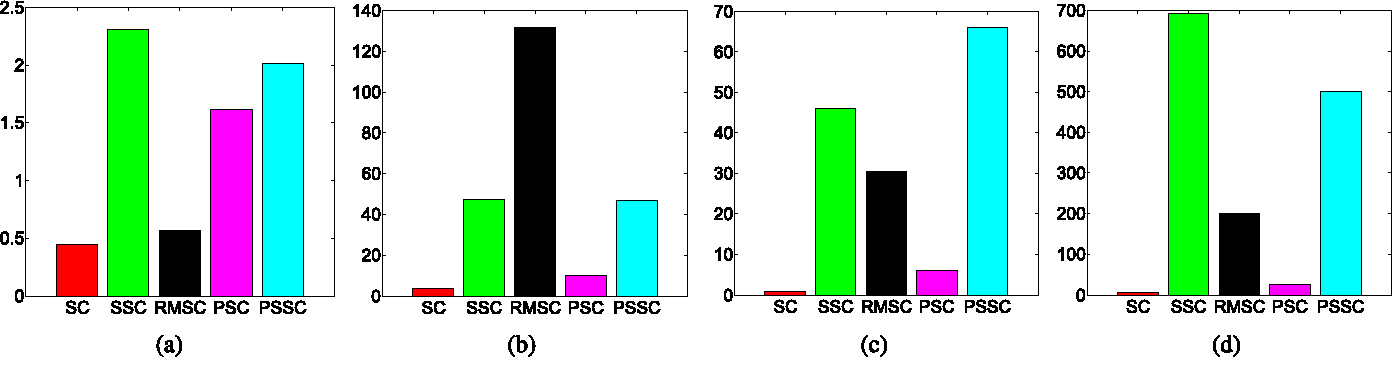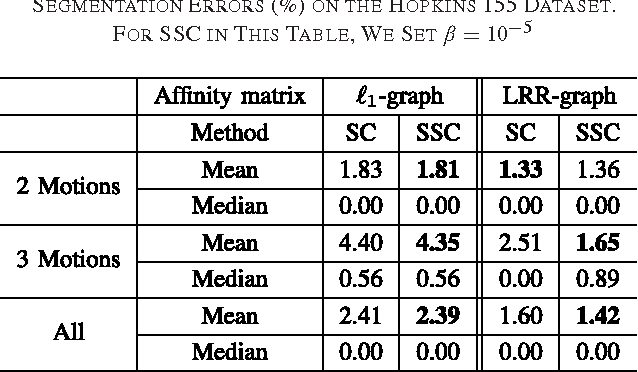Spectral Clustering (SC) is one of the most widely used methods for data clustering. It first finds a low-dimensonal embedding $U$ of data by computing the eigenvectors of the normalized Laplacian matrix, and then performs k-means on $U^\top$ to get the final clustering result. In this work, we observe that, in the ideal case, $UU^\top$ should be block diagonal and thus sparse. Therefore we propose the Sparse Spectral Clustering (SSC) method which extends SC with sparse regularization on $UU^\top$. To address the computational issue of the nonconvex SSC model, we propose a novel convex relaxation of SSC based on the convex hull of the fixed rank projection matrices. Then the convex SSC model can be efficiently solved by the Alternating Direction Method of \canyi{Multipliers} (ADMM). Furthermore, we propose the Pairwise Sparse Spectral Clustering (PSSC) which extends SSC to boost the clustering performance by using the multi-view information of data. Experimental comparisons with several baselines on real-world datasets testify to the efficacy of our proposed methods.

* IEEE Transactions on Image Processing (TIP), vol. 25, pp. 2833-2843, 2016
Click to Read Paper
In this paper we propose a new method to get the specified network parameters through one time feed-forward propagation of the meta networks and explore the application to neural style transfer. Recent works on style transfer typically need to train image transformation networks for every new style, and the style is encoded in the network parameters by enormous iterations of stochastic gradient descent. To tackle these issues, we build a meta network which takes in the style image and produces a corresponding image transformations network directly. Compared with optimization-based methods for every style, our meta networks can handle an arbitrary new style within $19ms$ seconds on one modern GPU card. The fast image transformation network generated by our meta network is only 449KB, which is capable of real-time executing on a mobile device. We also investigate the manifold of the style transfer networks by operating the hidden features from meta networks. Experiments have well validated the effectiveness of our method. Code and trained models has been released https://github.com/FalongShen/styletransfer.

Click to Read Paper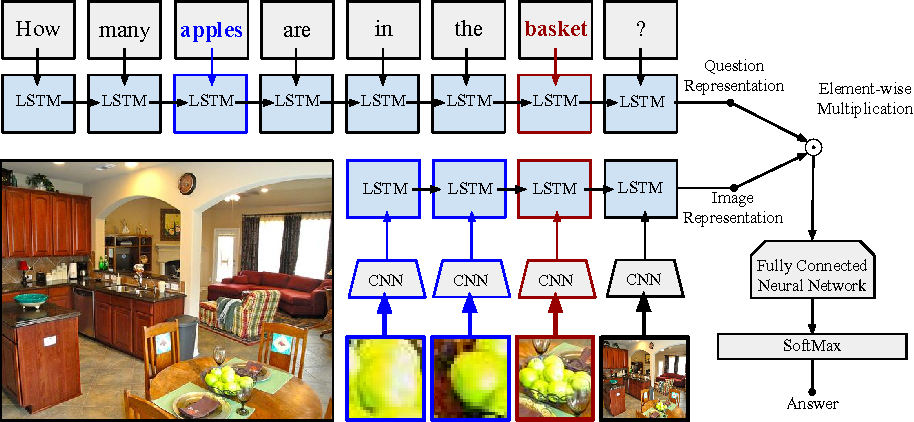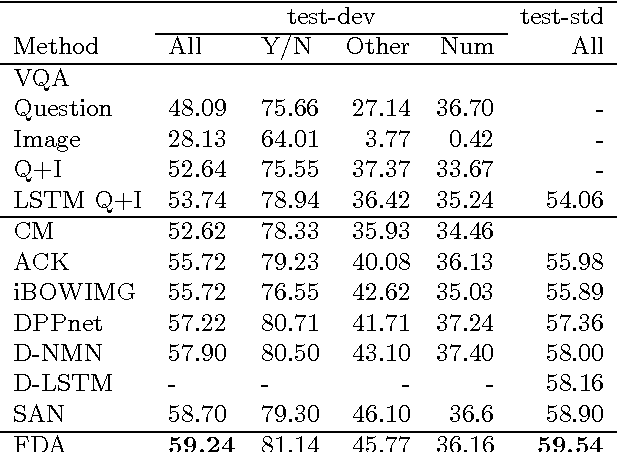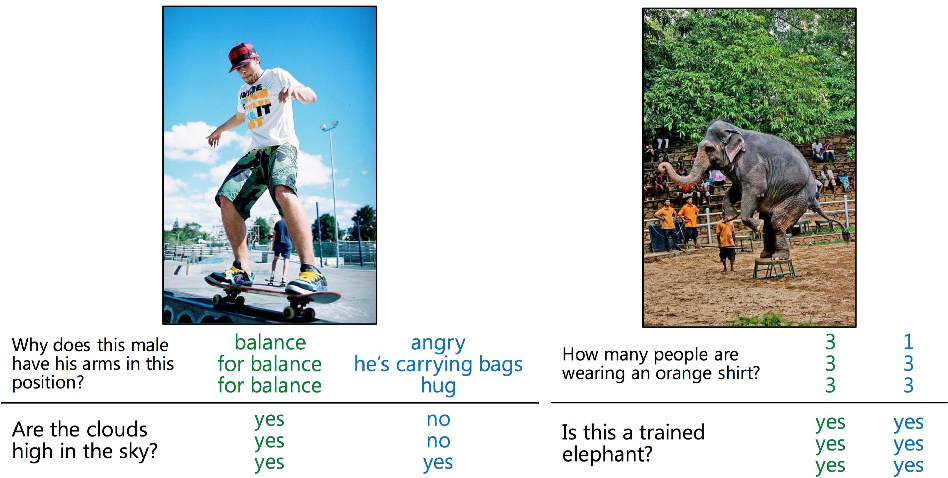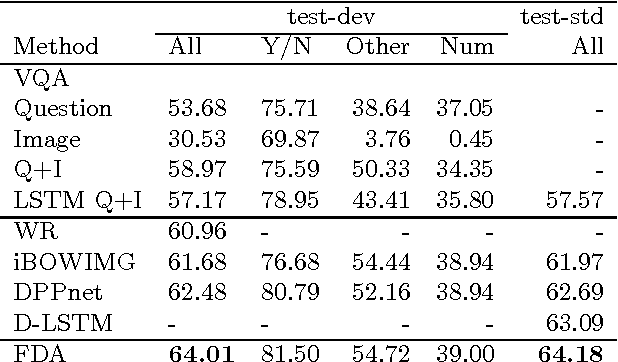Visual Question and Answering (VQA) problems are attracting increasing interest from multiple research disciplines. Solving VQA problems requires techniques from both computer vision for understanding the visual contents of a presented image or video, as well as the ones from natural language processing for understanding semantics of the question and generating the answers. Regarding visual content modeling, most of existing VQA methods adopt the strategy of extracting global features from the image or video, which inevitably fails in capturing fine-grained information such as spatial configuration of multiple objects. Extracting features from auto-generated regions -- as some region-based image recognition methods do -- cannot essentially address this problem and may introduce some overwhelming irrelevant features with the question. In this work, we propose a novel Focused Dynamic Attention (FDA) model to provide better aligned image content representation with proposed questions. Being aware of the key words in the question, FDA employs off-the-shelf object detector to identify important regions and fuse the information from the regions and global features via an LSTM unit. Such question-driven representations are then combined with question representation and fed into a reasoning unit for generating the answers. Extensive evaluation on a large-scale benchmark dataset, VQA, clearly demonstrate the superior performance of FDA over well-established baselines.

* Submitted to ECCV 2016
Click to Read Paper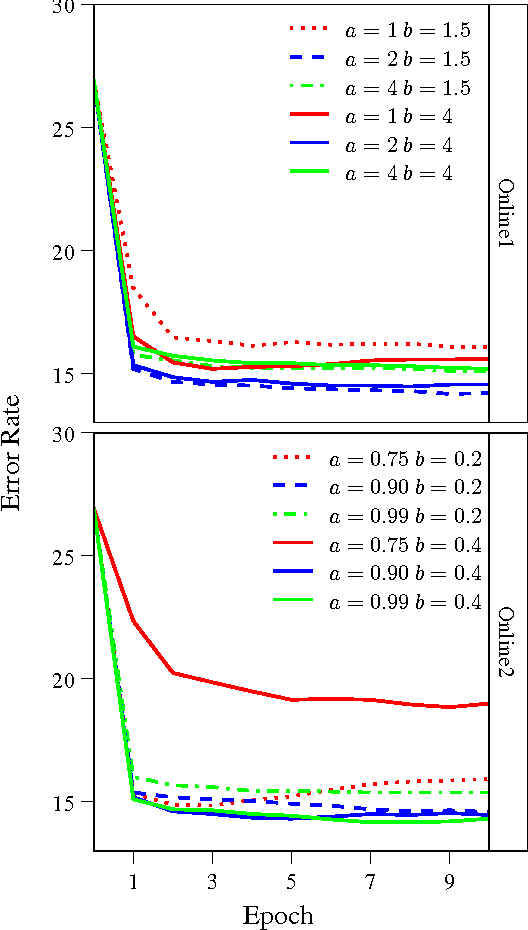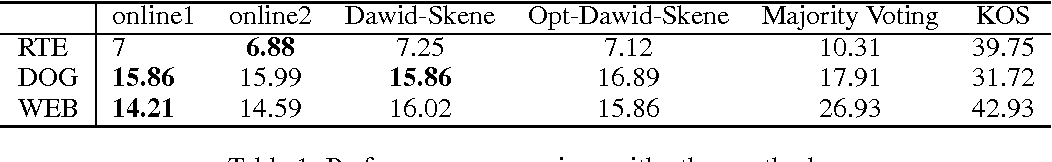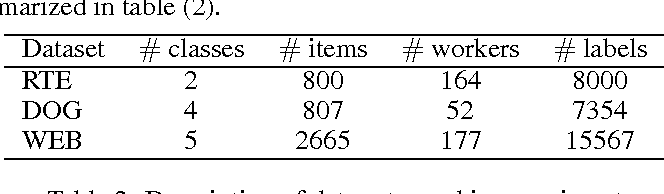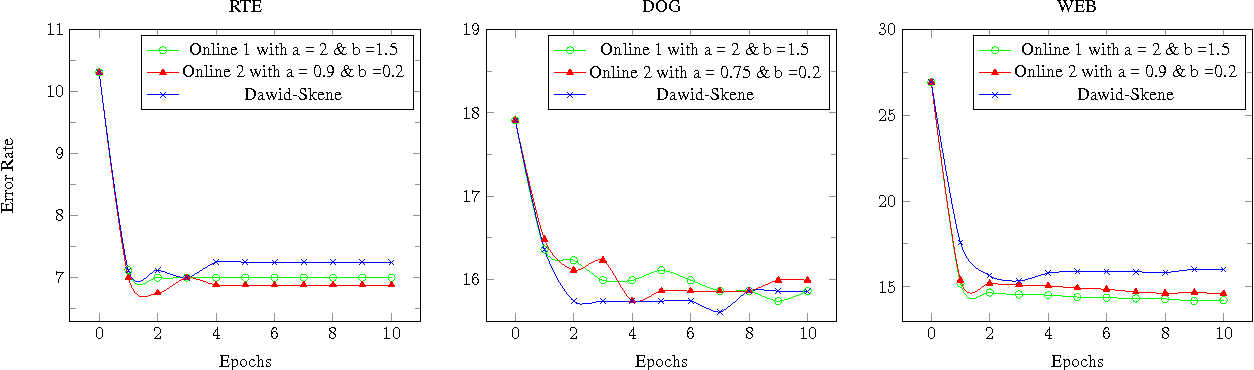With the success of modern internet based platform, such as Amazon Mechanical Turk, it is now normal to collect a large number of hand labeled samples from non-experts. The Dawid- Skene algorithm, which is based on Expectation- Maximization update, has been widely used for inferring the true labels from noisy crowdsourced labels. However, Dawid-Skene scheme requires all the data to perform each EM iteration, and can be infeasible for streaming data or large scale data. In this paper, we provide an online version of Dawid- Skene algorithm that only requires one data frame for each iteration. Further, we prove that under mild conditions, the online Dawid-Skene scheme with projection converges to a stationary point of the marginal log-likelihood of the observed data. Our experiments demonstrate that the online Dawid- Skene scheme achieves state of the art performance comparing with other methods based on the Dawid- Skene scheme.

Click to Read Paper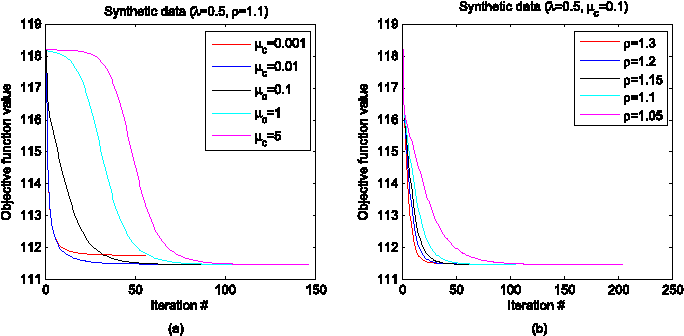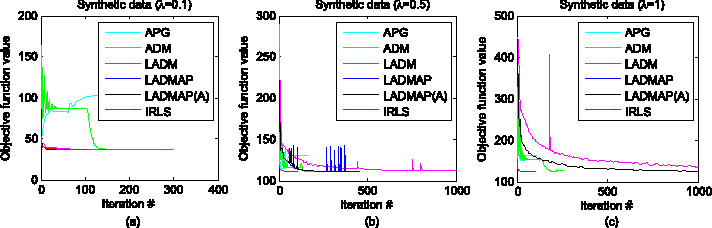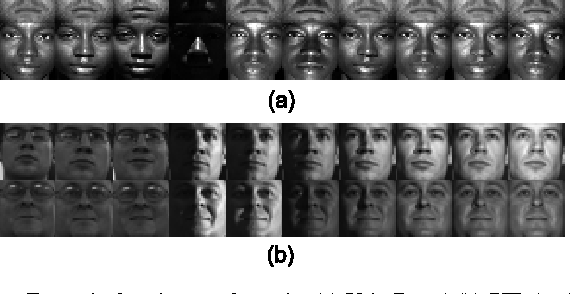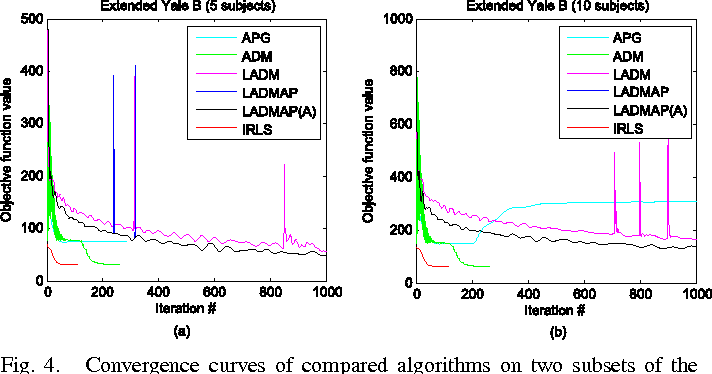This work presents a general framework for solving the low rank and/or sparse matrix minimization problems, which may involve multiple non-smooth terms. The Iteratively Reweighted Least Squares (IRLS) method is a fast solver, which smooths the objective function and minimizes it by alternately updating the variables and their weights. However, the traditional IRLS can only solve a sparse only or low rank only minimization problem with squared loss or an affine constraint. This work generalizes IRLS to solve joint/mixed low rank and sparse minimization problems, which are essential formulations for many tasks. As a concrete example, we solve the Schatten-$p$ norm and $\ell_{2,q}$-norm regularized Low-Rank Representation (LRR) problem by IRLS, and theoretically prove that the derived solution is a stationary point (globally optimal if $p,q\geq1$). Our convergence proof of IRLS is more general than previous one which depends on the special properties of the Schatten-$p$ norm and $\ell_{2,q}$-norm. Extensive experiments on both synthetic and real data sets demonstrate that our IRLS is much more efficient.

* IEEE Transactions on Image Processing 2015
Click to Read Paper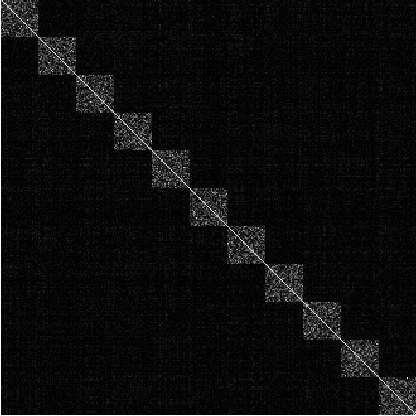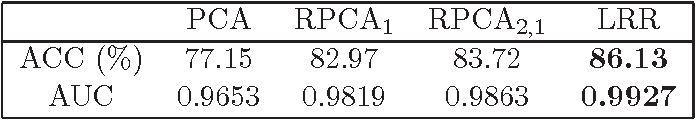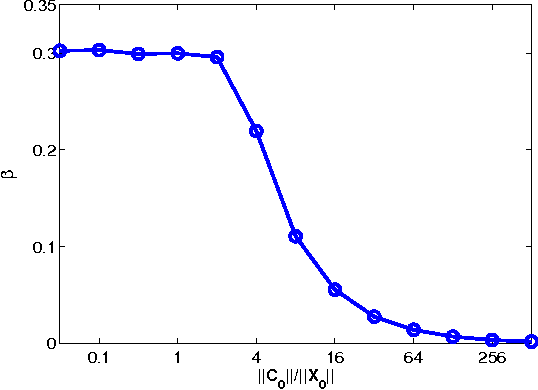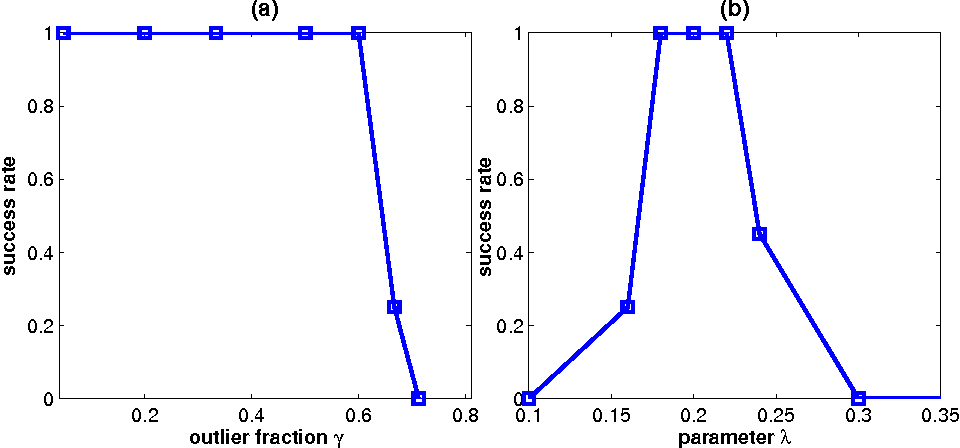In this work, we address the following matrix recovery problem: suppose we are given a set of data points containing two parts, one part consists of samples drawn from a union of multiple subspaces and the other part consists of outliers. We do not know which data points are outliers, or how many outliers there are. The rank and number of the subspaces are unknown either. Can we detect the outliers and segment the samples into their right subspaces, efficiently and exactly? We utilize a so-called {\em Low-Rank Representation} (LRR) method to solve this problem, and prove that under mild technical conditions, any solution to LRR exactly recovers the row space of the samples and detect the outliers as well. Since the subspace membership is provably determined by the row space, this further implies that LRR can perform exact subspace segmentation and outlier detection, in an efficient way.

* Proceedings of the Fifteenth International Conference on Artificial Intelligence and Statistics, AISTATS 2012
Click to Read Paper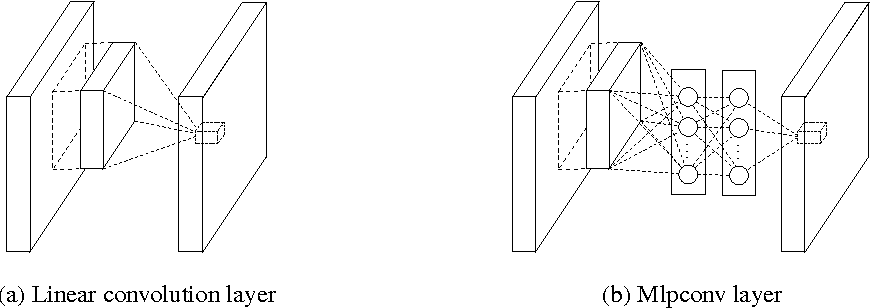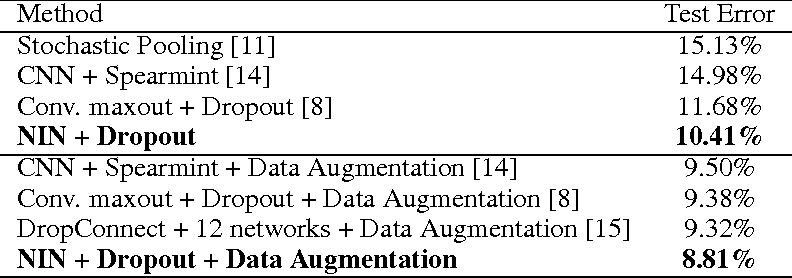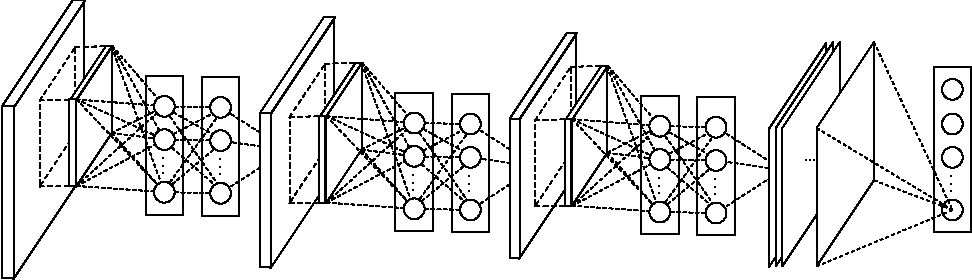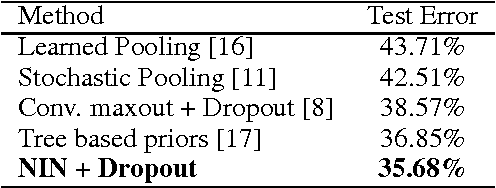We propose a novel deep network structure called "Network In Network" (NIN) to enhance model discriminability for local patches within the receptive field. The conventional convolutional layer uses linear filters followed by a nonlinear activation function to scan the input. Instead, we build micro neural networks with more complex structures to abstract the data within the receptive field. We instantiate the micro neural network with a multilayer perceptron, which is a potent function approximator. The feature maps are obtained by sliding the micro networks over the input in a similar manner as CNN; they are then fed into the next layer. Deep NIN can be implemented by stacking mutiple of the above described structure. With enhanced local modeling via the micro network, we are able to utilize global average pooling over feature maps in the classification layer, which is easier to interpret and less prone to overfitting than traditional fully connected layers. We demonstrated the state-of-the-art classification performances with NIN on CIFAR-10 and CIFAR-100, and reasonable performances on SVHN and MNIST datasets.

* 10 pages, 4 figures, for iclr2014
Click to Read Paper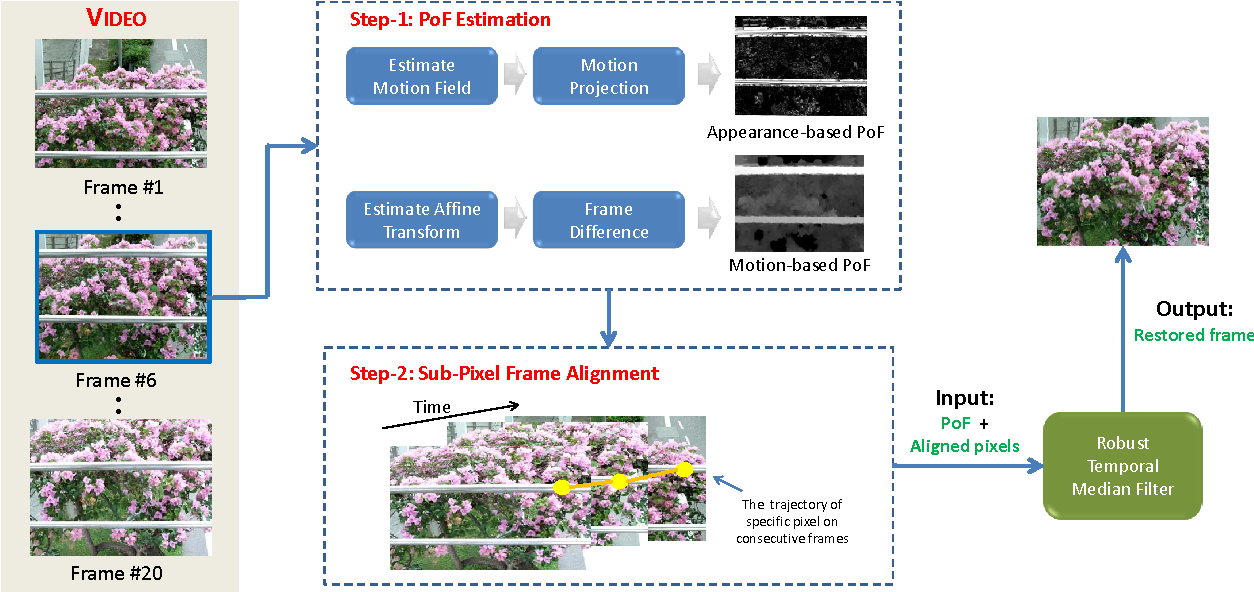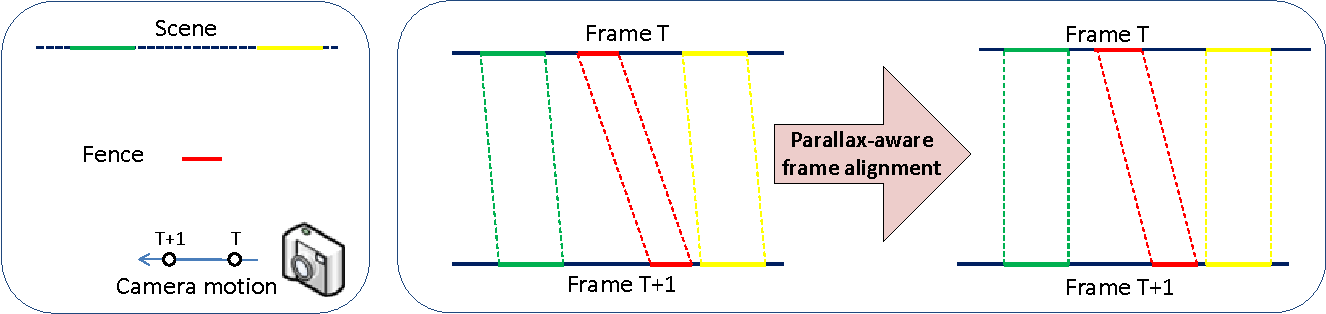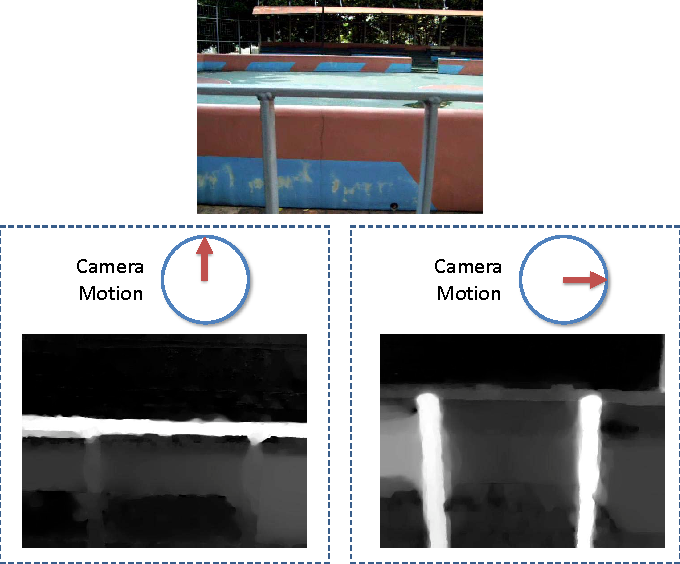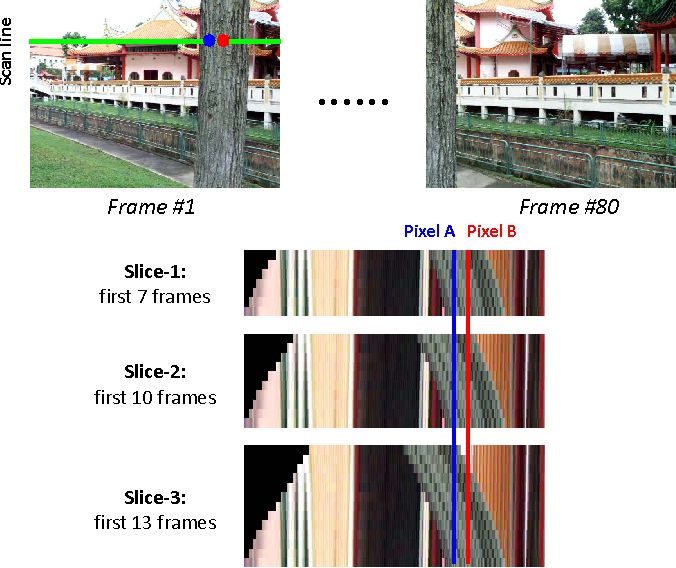This paper describes and provides an initial solution to a novel video editing task, i.e., video de-fencing. It targets automatic restoration of the video clips that are corrupted by fence-like occlusions during capture. Our key observation lies in the visual parallax between fences and background scenes, which is caused by the fact that the former are typically closer to the camera. Unlike in traditional image inpainting, fence-occluded pixels in the videos tend to appear later in the temporal dimension and are therefore recoverable via optimized pixel selection from relevant frames. To eventually produce fence-free videos, major challenges include cross-frame sub-pixel image alignment under diverse scene depth, and "correct" pixel selection that is robust to dominating fence pixels. Several novel tools are developed in this paper, including soft fence detection, weighted truncated optical flow method and robust temporal median filter. The proposed algorithm is validated on several real-world video clips with fences.

* To appear in IEEE transactions on Circuits and Systems for Video Technology (T-CSVT)
Click to Read Paper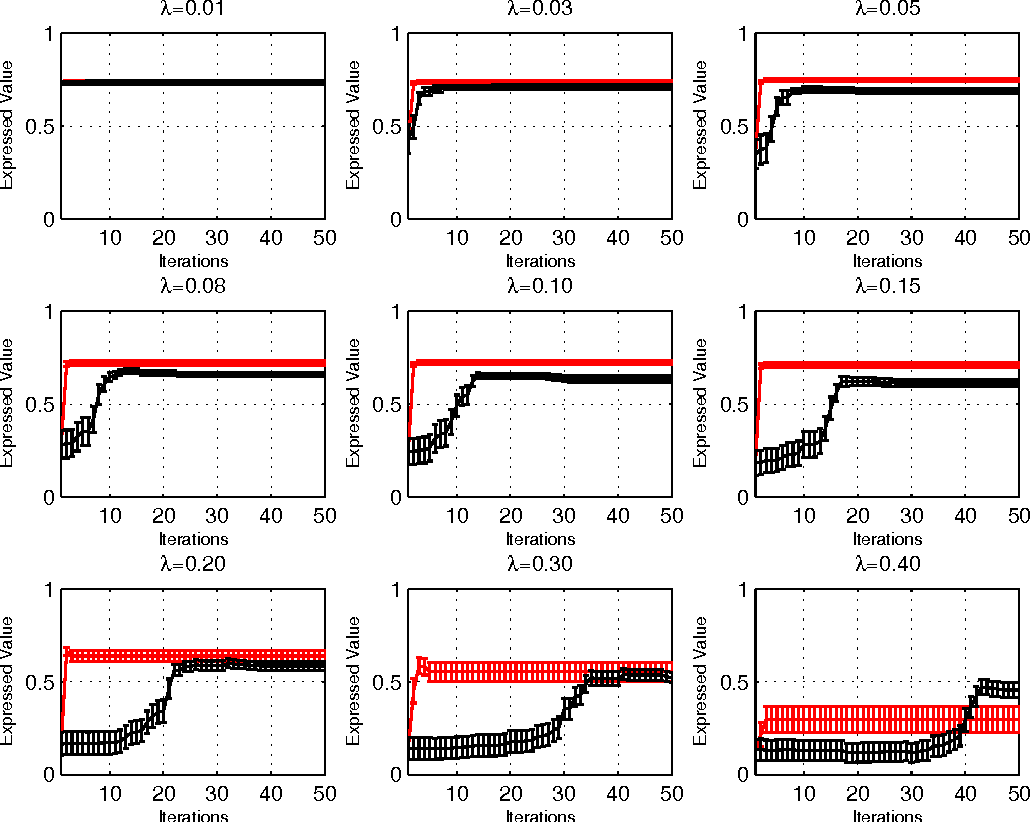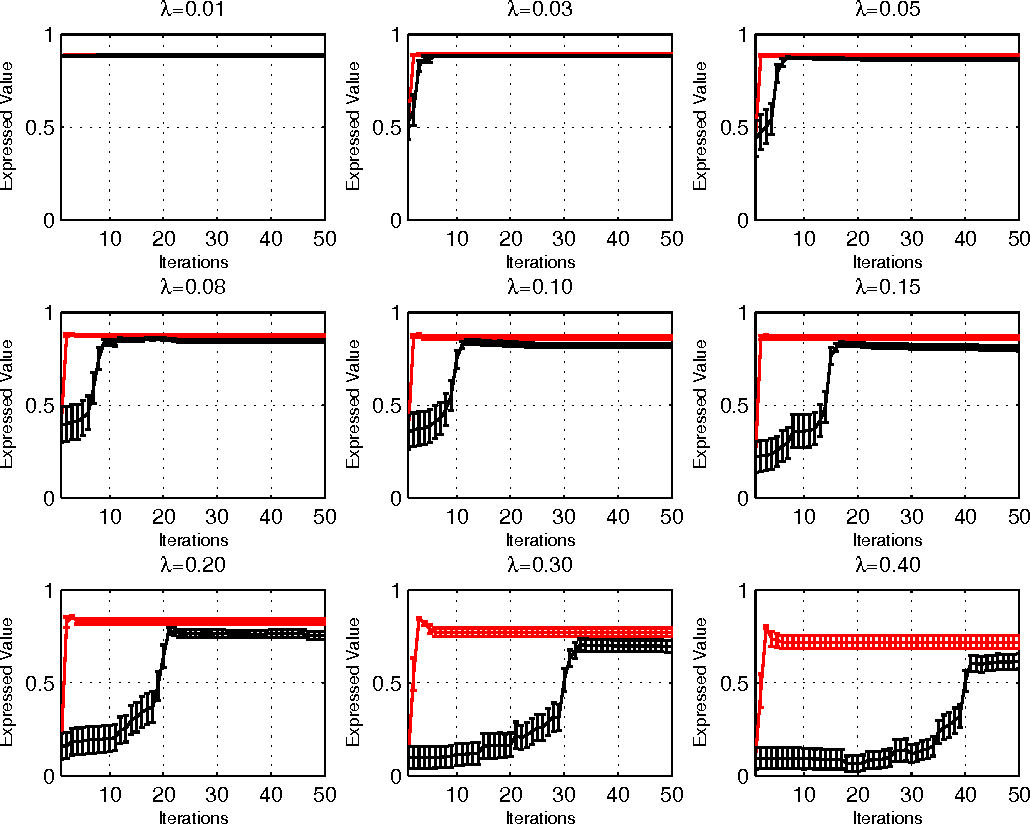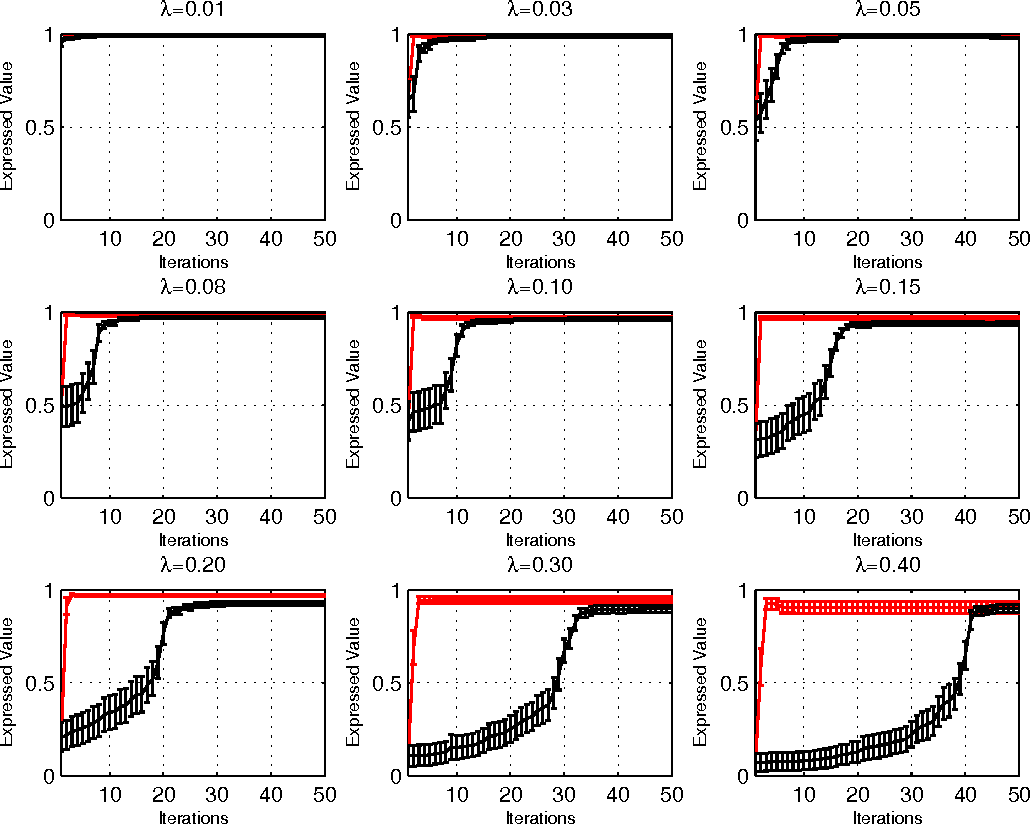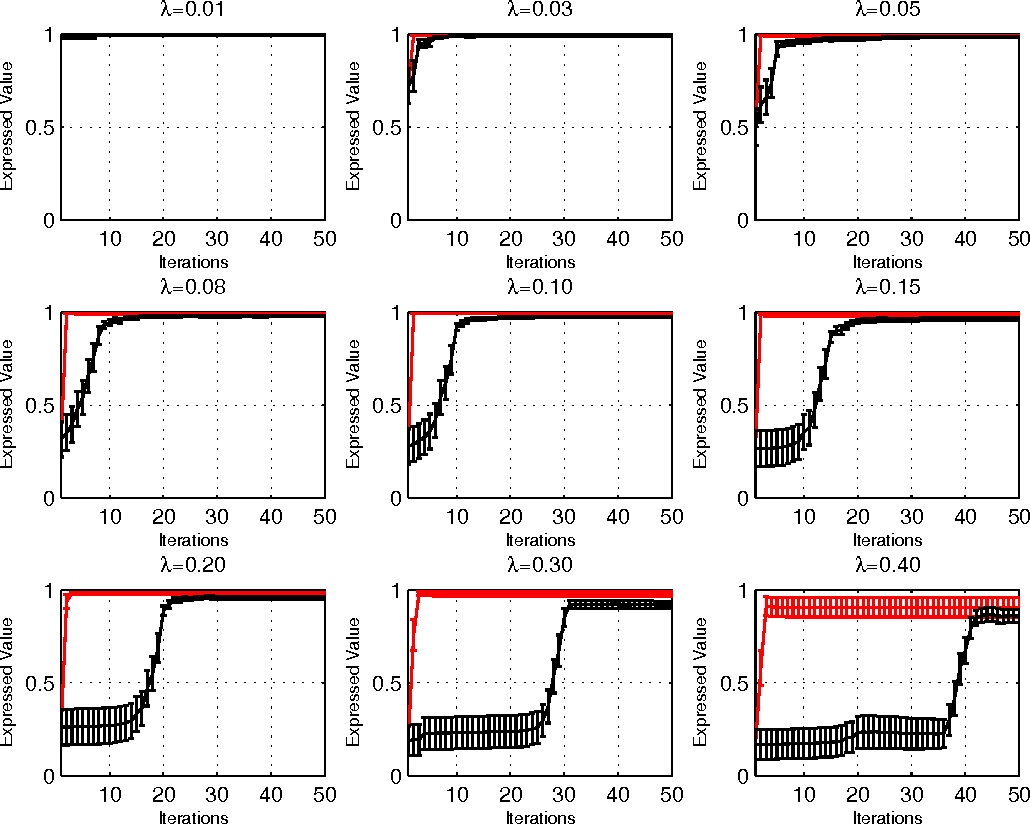We consider principal component analysis for contaminated data-set in the high dimensional regime, where the dimensionality of each observation is comparable or even more than the number of observations. We propose a deterministic high-dimensional robust PCA algorithm which inherits all theoretical properties of its randomized counterpart, i.e., it is tractable, robust to contaminated points, easily kernelizable, asymptotic consistent and achieves maximal robustness -- a breakdown point of 50%. More importantly, the proposed method exhibits significantly better computational efficiency, which makes it suitable for large-scale real applications.

* ICML2012
Click to Read Paper
It is an efficient and effective strategy to utilize the nuclear norm approximation to learn low-rank matrices, which arise frequently in machine learning and computer vision. So the exploration of nuclear norm minimization problems is gaining much attention recently. In this paper we shall prove that the following Low-Rank Representation (LRR) \cite{icml_2010_lrr,lrr_extention} problem: {eqnarray*} \min_{Z} \norm{Z}_*, & {s.t.,} & X=AZ, {eqnarray*} has a unique and closed-form solution, where $X$ and $A$ are given matrices. The proof is based on proving a lemma that allows us to get closed-form solutions to a category of nuclear norm minimization problems.

* NIPS Workshop on Low-Rank Methods for Large-Scale Machine Learning, 2010
Click to Read Paper
In this paper, we propose a non-convex formulation to recover the authentic structure from the corrupted real data. Typically, the specific structure is assumed to be low rank, which holds for a wide range of data, such as images and videos. Meanwhile, the corruption is assumed to be sparse. In the literature, such a problem is known as Robust Principal Component Analysis (RPCA), which usually recovers the low rank structure by approximating the rank function with a nuclear norm and penalizing the error by an $\ell_1$-norm. Although RPCA is a convex formulation and can be solved effectively, the introduced norms are not tight approximations, which may cause the solution to deviate from the authentic one. Therefore, we consider here a non-convex relaxation, consisting of a Schatten-$p$ norm and an $\ell_q$-norm that promote low rank and sparsity respectively. We derive a proximal iteratively reweighted algorithm (PIRA) to solve the problem. Our algorithm is based on an alternating direction method of multipliers, where in each iteration we linearize the underlying objective function that allows us to have a closed form solution. We demonstrate that solutions produced by the linearized approximation always converge and have a tighter approximation than the convex counterpart. Experimental results on benchmarks show encouraging results of our approach.

* Pattern Recognition, 2015
Click to Read Paper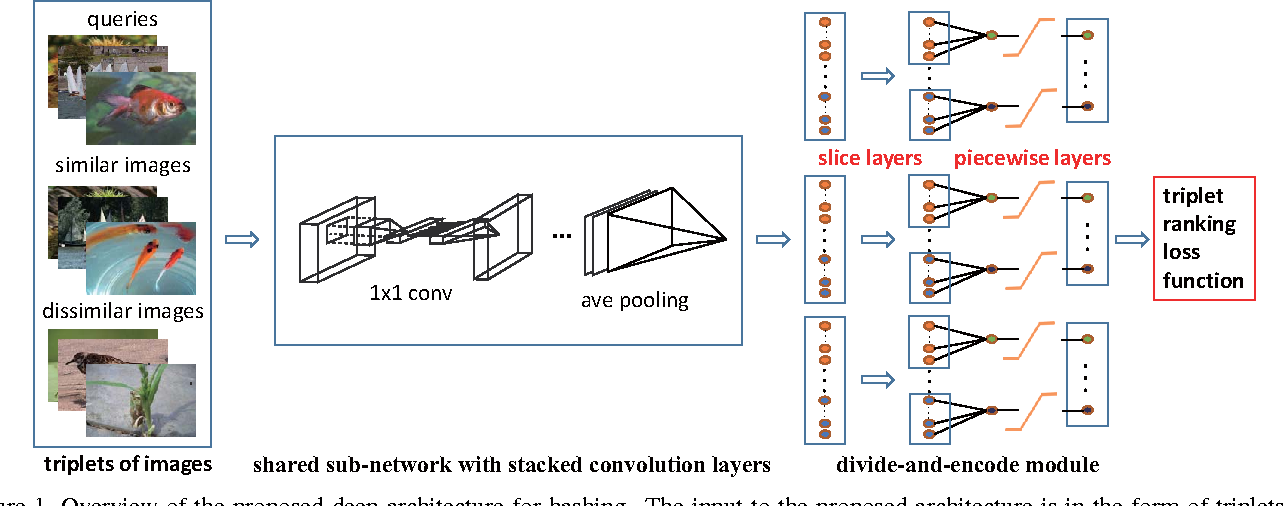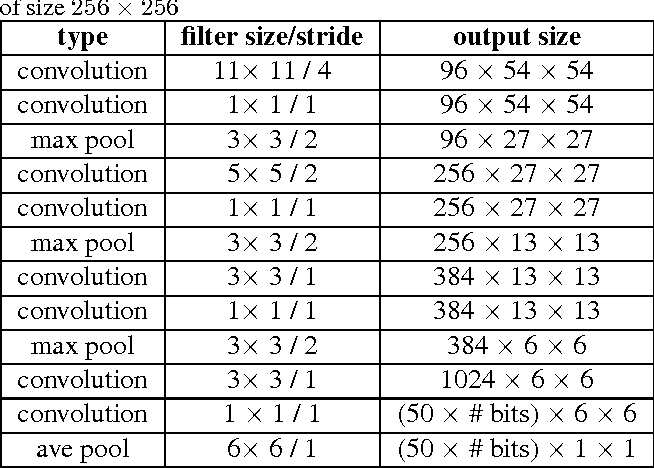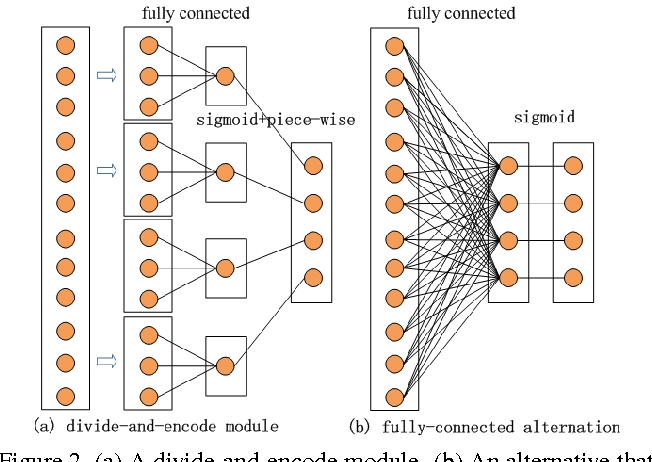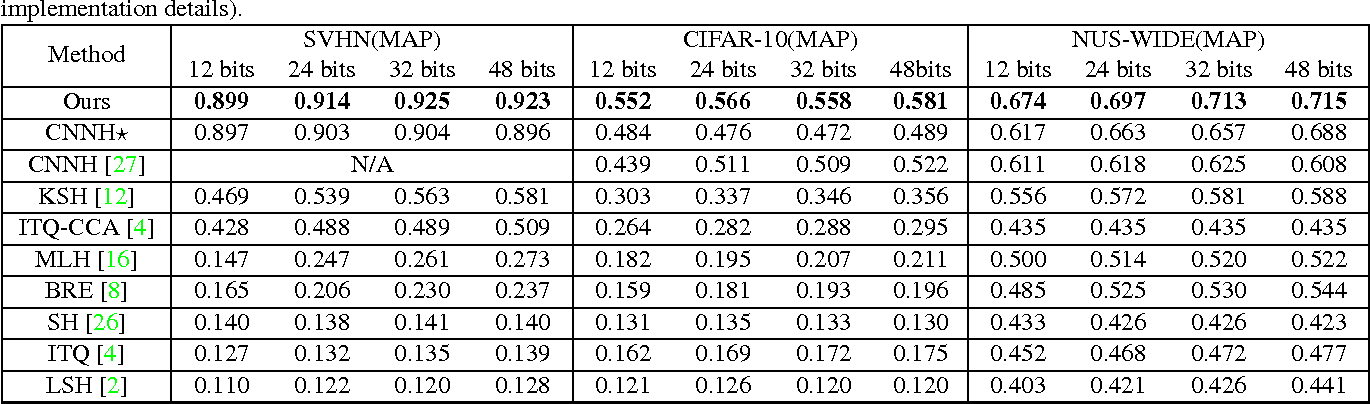Similarity-preserving hashing is a widely-used method for nearest neighbour search in large-scale image retrieval tasks. For most existing hashing methods, an image is first encoded as a vector of hand-engineering visual features, followed by another separate projection or quantization step that generates binary codes. However, such visual feature vectors may not be optimally compatible with the coding process, thus producing sub-optimal hashing codes. In this paper, we propose a deep architecture for supervised hashing, in which images are mapped into binary codes via carefully designed deep neural networks. The pipeline of the proposed deep architecture consists of three building blocks: 1) a sub-network with a stack of convolution layers to produce the effective intermediate image features; 2) a divide-and-encode module to divide the intermediate image features into multiple branches, each encoded into one hash bit; and 3) a triplet ranking loss designed to characterize that one image is more similar to the second image than to the third one. Extensive evaluations on several benchmark image datasets show that the proposed simultaneous feature learning and hash coding pipeline brings substantial improvements over other state-of-the-art supervised or unsupervised hashing methods.

* This paper has been accepted to IEEE International Conference on Pattern Recognition and Computer Vision (CVPR), 2015
Click to Read Paper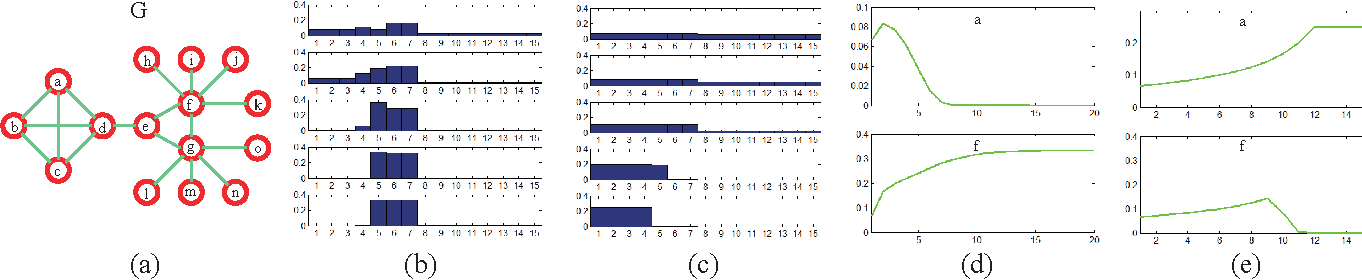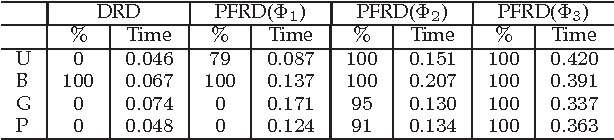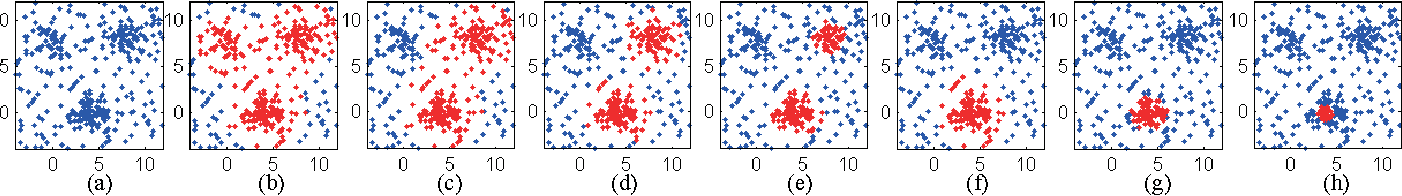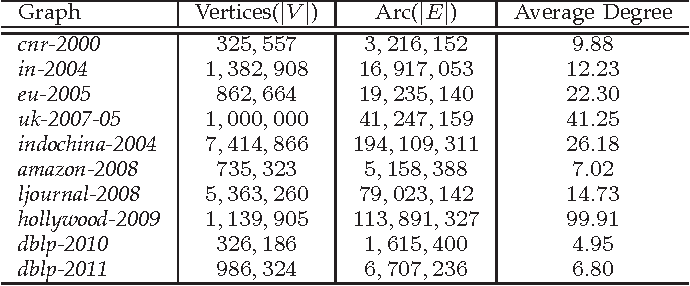In this paper, we propose a path following replicator dynamic, and investigate its potentials in uncovering the underlying cluster structure of a graph. The proposed dynamic is a generalization of the discrete replicator dynamic. The replicator dynamic has been successfully used to extract dense clusters of graphs; however, it is often sensitive to the degree distribution of a graph, and usually biased by vertices with large degrees, thus may fail to detect the densest cluster. To overcome this problem, we introduce a dynamic parameter, called path parameter, into the evolution process. The path parameter can be interpreted as the maximal possible probability of a current cluster containing a vertex, and it monotonically increases as evolution process proceeds. By limiting the maximal probability, the phenomenon of some vertices dominating the early stage of evolution process is suppressed, thus making evolution process more robust. To solve the optimization problem with a fixed path parameter, we propose an efficient fixed point algorithm. The time complexity of the path following replicator dynamic is only linear in the number of edges of a graph, thus it can analyze graphs with millions of vertices and tens of millions of edges on a common PC in a few minutes. Besides, it can be naturally generalized to hypergraph and graph with edges of different orders. We apply it to four important problems: maximum clique problem, densest k-subgraph problem, structure fitting, and discovery of high-density regions. The extensive experimental results clearly demonstrate its advantages, in terms of robustness, scalability and flexility.

Click to Read Paper
The recent proposed Tensor Nuclear Norm (TNN) [Lu et al., 2016; 2018a] is an interesting convex penalty induced by the tensor SVD [Kilmer and Martin, 2011]. It plays a similar role as the matrix nuclear norm which is the convex surrogate of the matrix rank. Considering that the TNN based Tensor Robust PCA [Lu et al., 2018a] is an elegant extension of Robust PCA with a similar tight recovery bound, it is natural to solve other low rank tensor recovery problems extended from the matrix cases. However, the extensions and proofs are generally tedious. The general atomic norm provides a unified view of low-complexity structures induced norms, e.g., the $\ell_1$-norm and nuclear norm. The sharp estimates of the required number of generic measurements for exact recovery based on the atomic norm are known in the literature. In this work, with a careful choice of the atomic set, we prove that TNN is a special atomic norm. Then by computing the Gaussian width of certain cone which is necessary for the sharp estimate, we achieve a simple bound for guaranteed low tubal rank tensor recovery from Gaussian measurements. Specifically, we show that by solving a TNN minimization problem, the underlying tensor of size $n_1\times n_2\times n_3$ with tubal rank $r$ can be exactly recovered when the given number of Gaussian measurements is $O(r(n_1+n_2-r)n_3)$. It is order optimal when comparing with the degrees of freedom $r(n_1+n_2-r)n_3$. Beyond the Gaussian mapping, we also give the recovery guarantee of tensor completion based on the uniform random mapping by TNN minimization. Numerical experiments verify our theoretical results.

* International Joint Conference on Artificial Intelligence (IJCAI), 2018
Click to Read Paper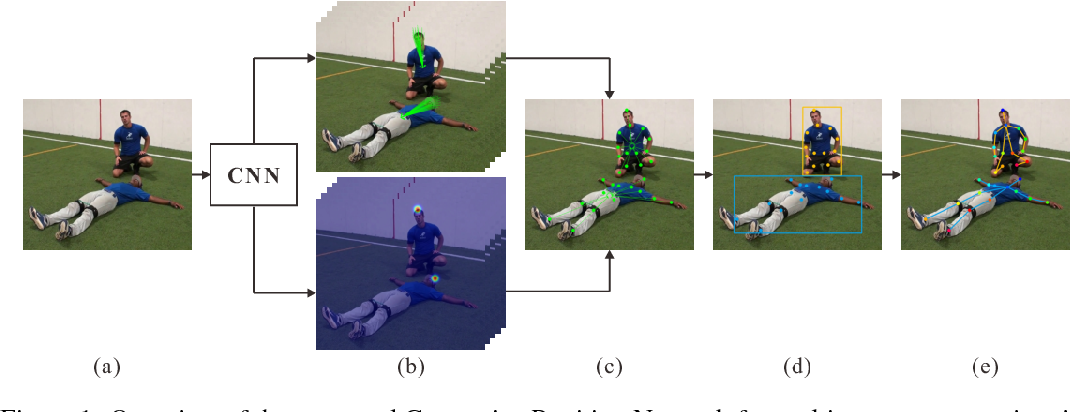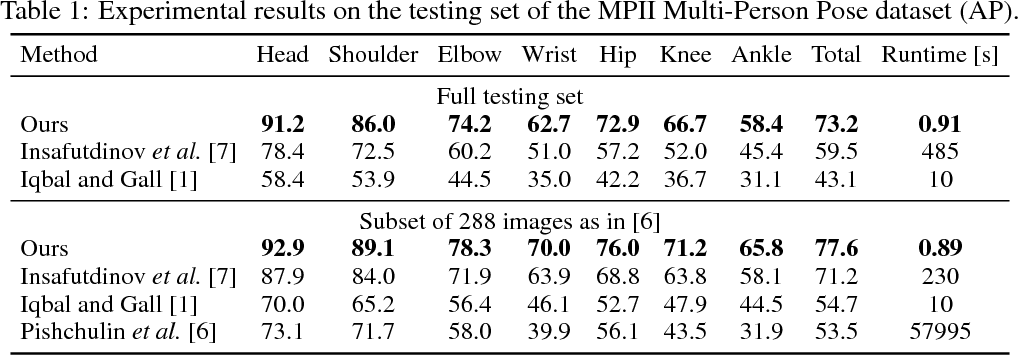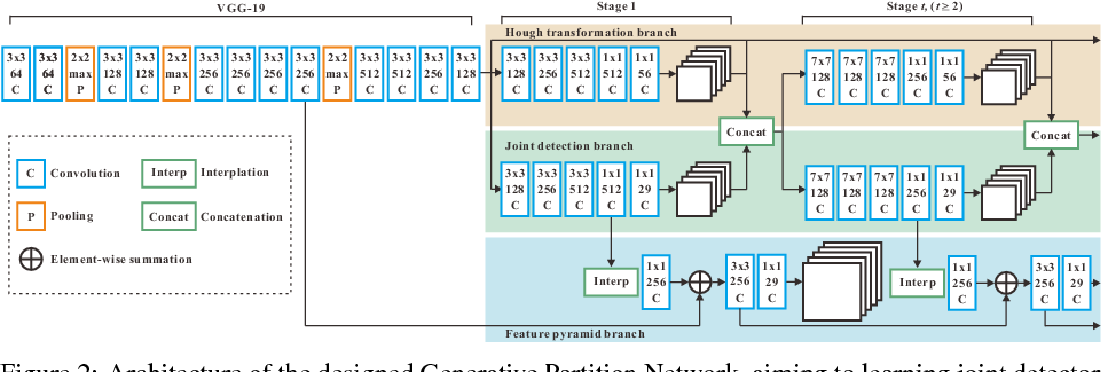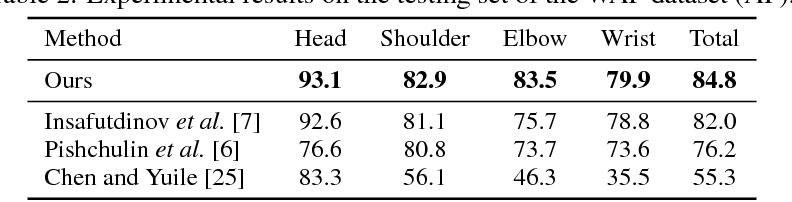This paper proposes a new Generative Partition Network (GPN) to address the challenging multi-person pose estimation problem. Different from existing models that are either completely top-down or bottom-up, the proposed GPN introduces a novel strategy--it generates partitions for multiple persons from their global joint candidates and infers instance-specific joint configurations simultaneously. The GPN is favorably featured by low complexity and high accuracy of joint detection and re-organization. In particular, GPN designs a generative model that performs one feed-forward pass to efficiently generate robust person detections with joint partitions, relying on dense regressions from global joint candidates in an embedding space parameterized by centroids of persons. In addition, GPN formulates the inference procedure for joint configurations of human poses as a graph partition problem, and conducts local optimization for each person detection with reliable global affinity cues, leading to complexity reduction and performance improvement. GPN is implemented with the Hourglass architecture as the backbone network to simultaneously learn joint detector and dense regressor. Extensive experiments on benchmarks MPII Human Pose Multi-Person, extended PASCAL-Person-Part, and WAF, show the efficiency of GPN with new state-of-the-art performance.

Click to Read Paper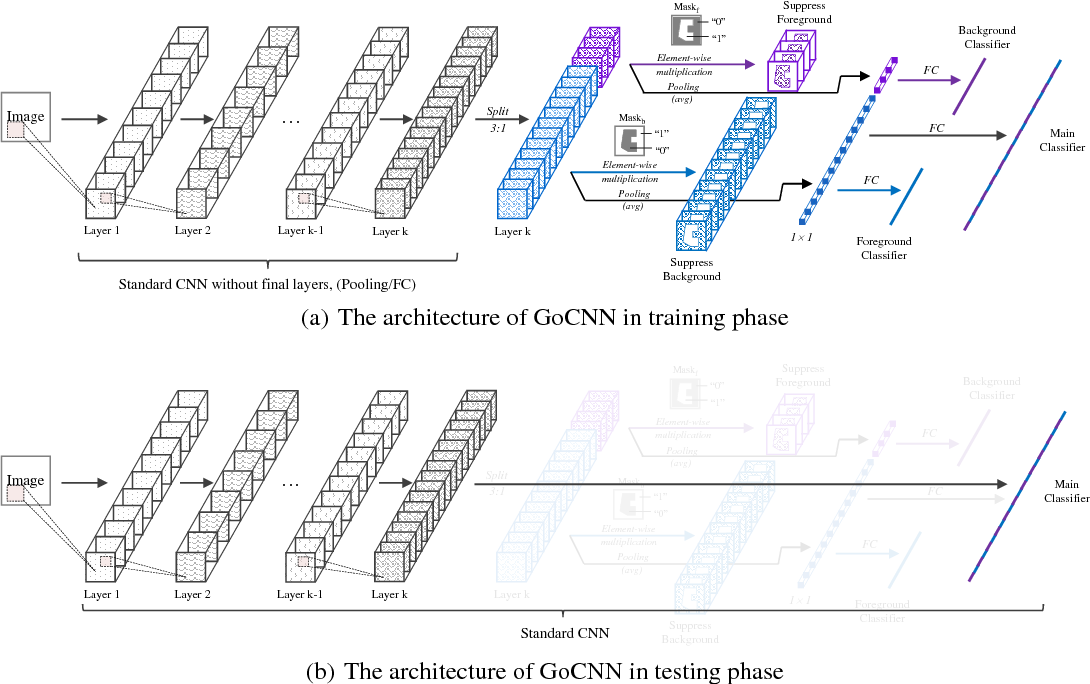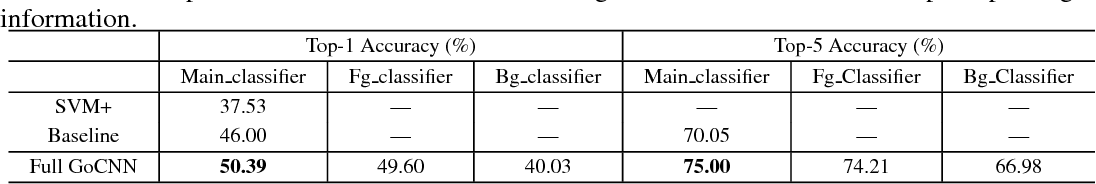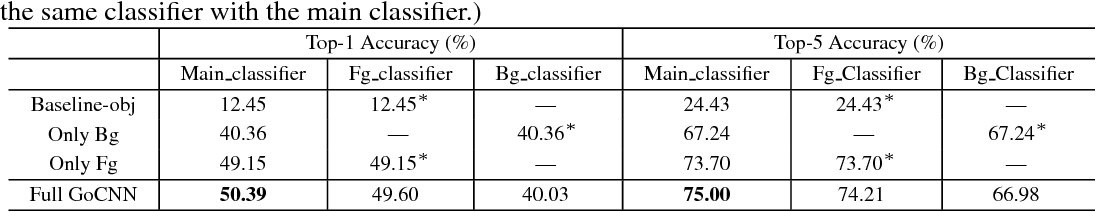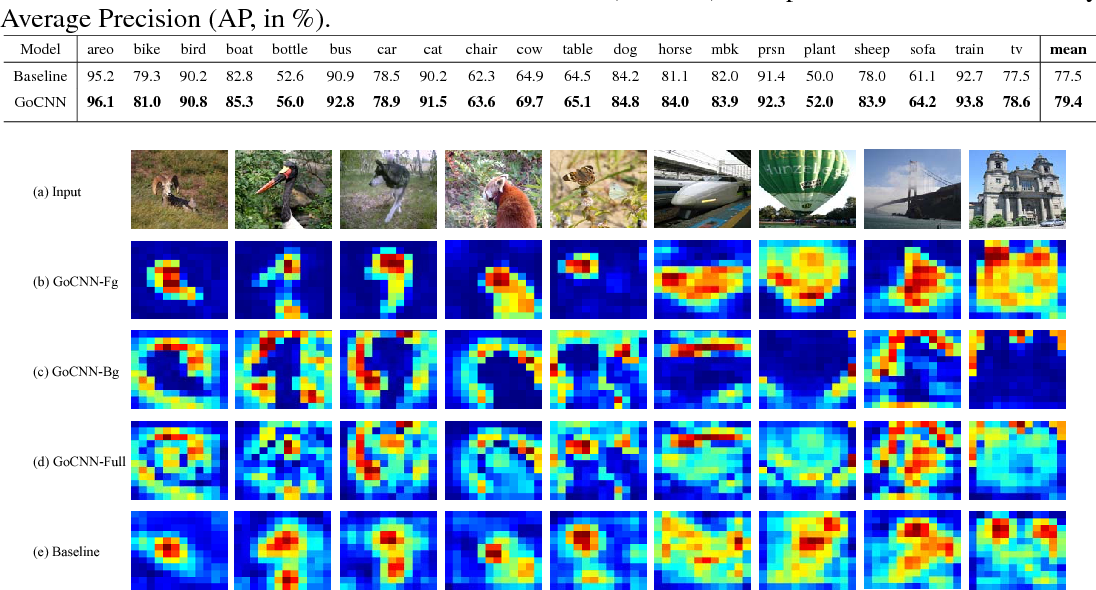Learning rich and diverse representations is critical for the performance of deep convolutional neural networks (CNNs). In this paper, we consider how to use privileged information to promote inherent diversity of a single CNN model such that the model can learn better representations and offer stronger generalization ability. To this end, we propose a novel group orthogonal convolutional neural network (GoCNN) that learns untangled representations within each layer by exploiting provided privileged information and enhances representation diversity effectively. We take image classification as an example where image segmentation annotations are used as privileged information during the training process. Experiments on two benchmark datasets -- ImageNet and PASCAL VOC -- clearly demonstrate the strong generalization ability of our proposed GoCNN model. On the ImageNet dataset, GoCNN improves the performance of state-of-the-art ResNet-152 model by absolute value of 1.2% while only uses privileged information of 10% of the training images, confirming effectiveness of GoCNN on utilizing available privileged knowledge to train better CNNs.

* Proceedings of the IJCAI-17
Click to Read Paper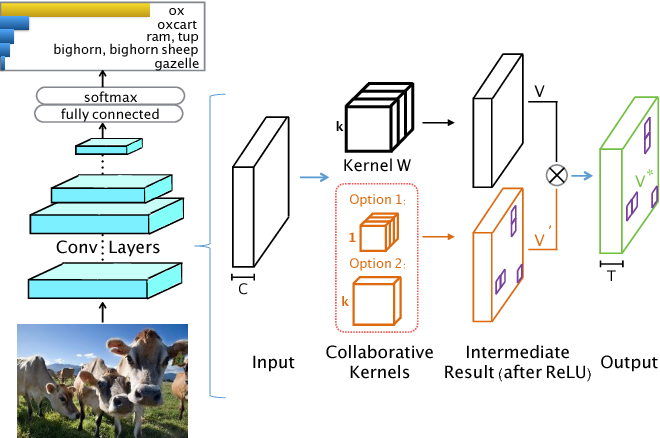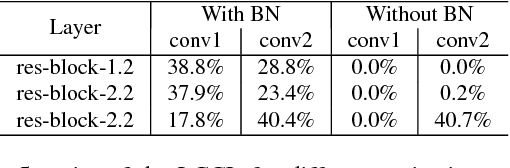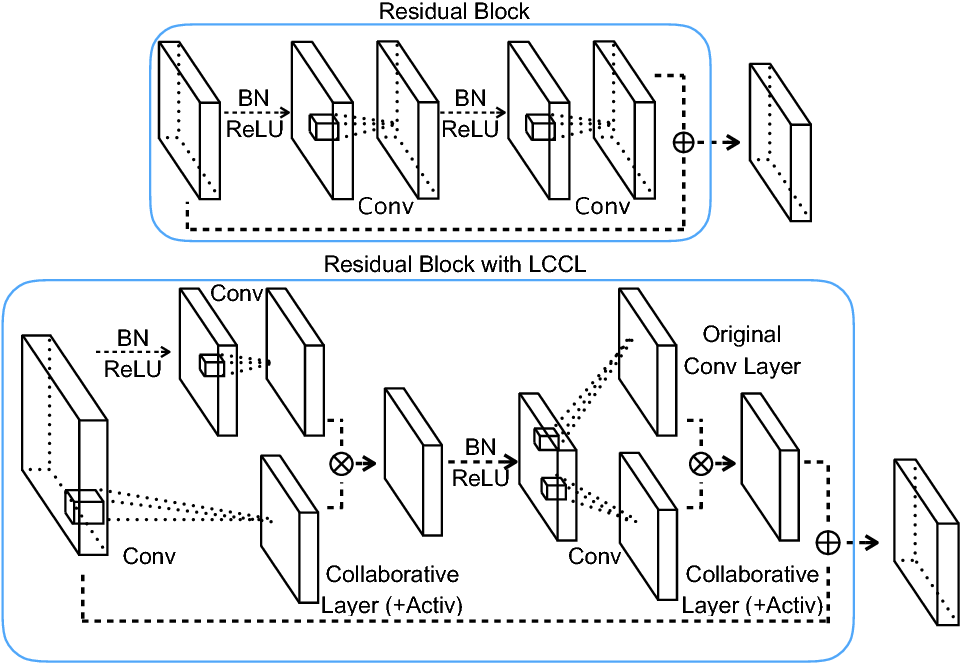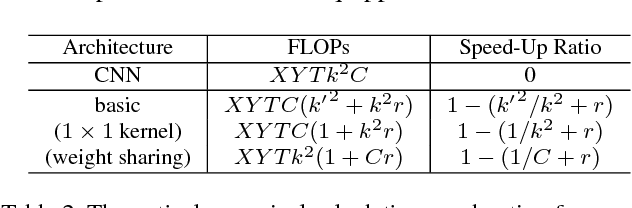In this paper, we present a novel and general network structure towards accelerating the inference process of convolutional neural networks, which is more complicated in network structure yet with less inference complexity. The core idea is to equip each original convolutional layer with another low-cost collaborative layer (LCCL), and the element-wise multiplication of the ReLU outputs of these two parallel layers produces the layer-wise output. The combined layer is potentially more discriminative than the original convolutional layer, and its inference is faster for two reasons: 1) the zero cells of the LCCL feature maps will remain zero after element-wise multiplication, and thus it is safe to skip the calculation of the corresponding high-cost convolution in the original convolutional layer, 2) LCCL is very fast if it is implemented as a 1*1 convolution or only a single filter shared by all channels. Extensive experiments on the CIFAR-10, CIFAR-100 and ILSCRC-2012 benchmarks show that our proposed network structure can accelerate the inference process by 32\% on average with negligible performance drop.

* This paper has been accepted by the IEEE CVPR 2017
Click to Read Paper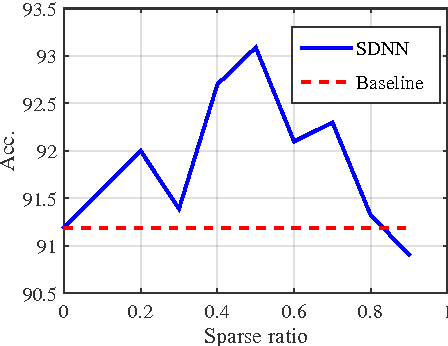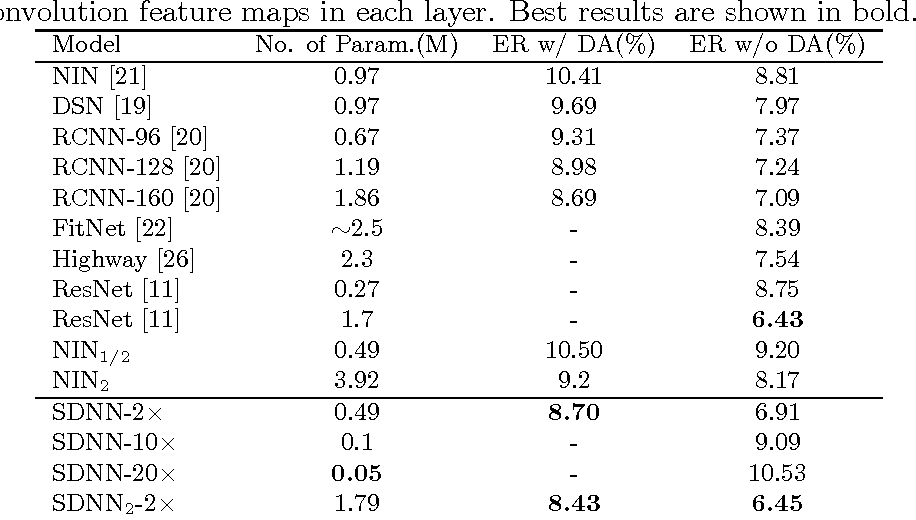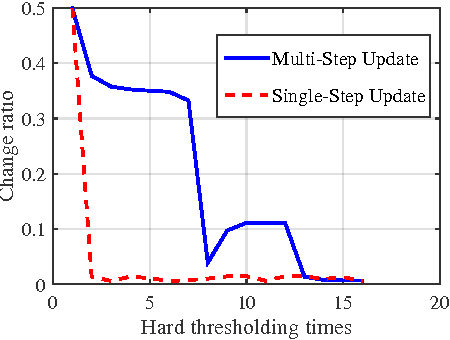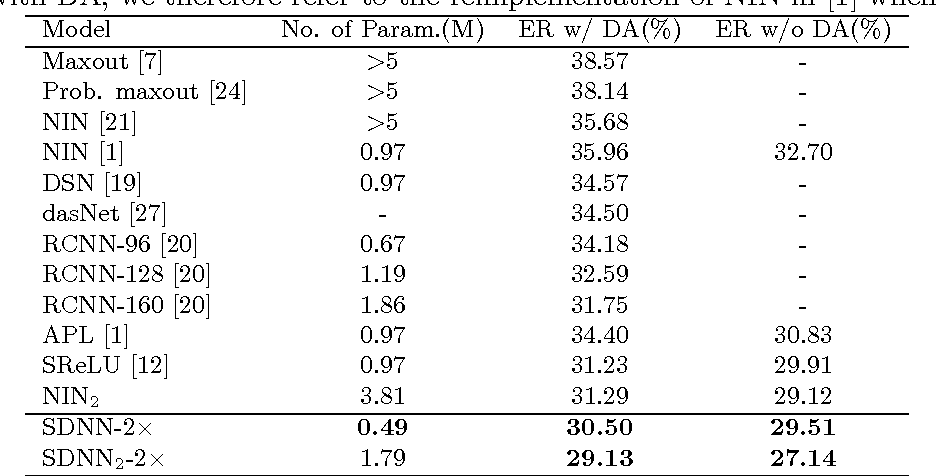Deep neural networks have achieved remarkable success in a wide range of practical problems. However, due to the inherent large parameter space, deep models are notoriously prone to overfitting and difficult to be deployed in portable devices with limited memory. In this paper, we propose an iterative hard thresholding (IHT) approach to train Skinny Deep Neural Networks (SDNNs). An SDNN has much fewer parameters yet can achieve competitive or even better performance than its full CNN counterpart. More concretely, the IHT approach trains an SDNN through following two alternative phases: (I) perform hard thresholding to drop connections with small activations and fine-tune the other significant filters; (II)~re-activate the frozen connections and train the entire network to improve its overall discriminative capability. We verify the superiority of SDNNs in terms of efficiency and classification performance on four benchmark object recognition datasets, including CIFAR-10, CIFAR-100, MNIST and ImageNet. Experimental results clearly demonstrate that IHT can be applied for training SDNN based on various CNN architectures such as NIN and AlexNet.

Click to Read Paper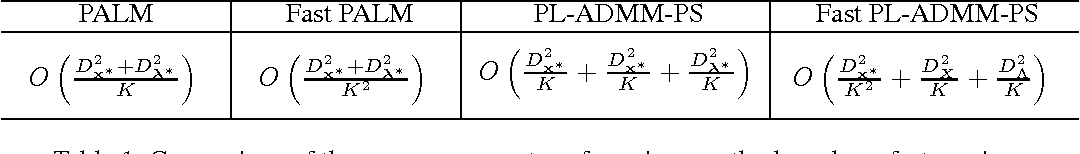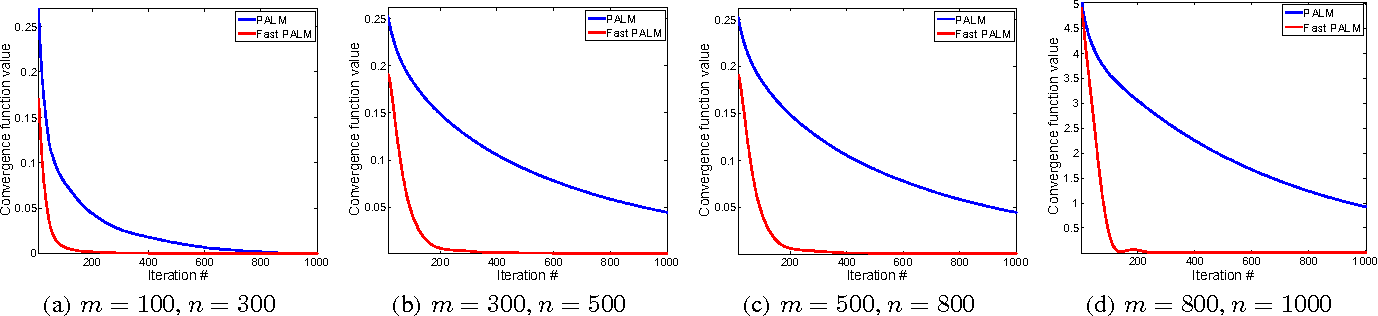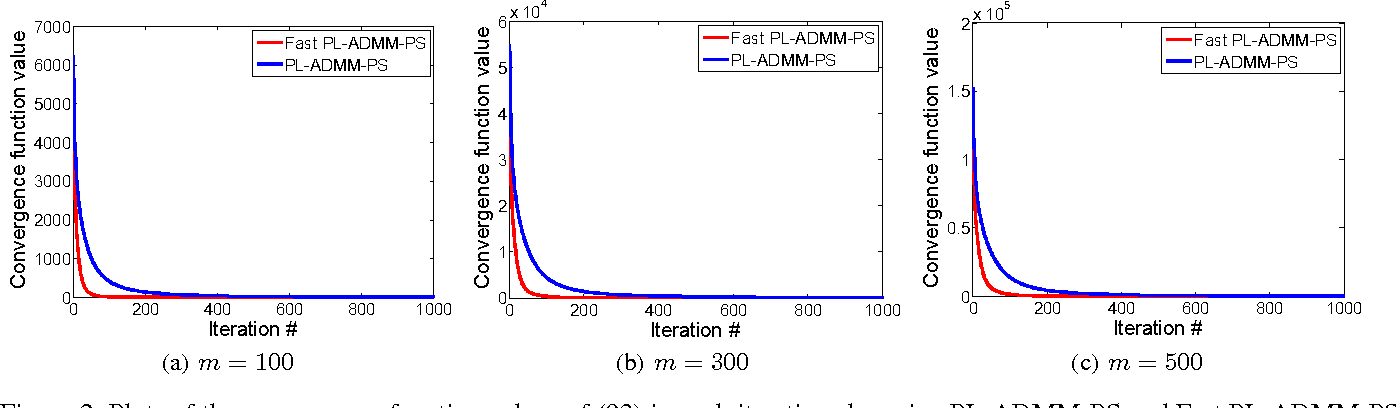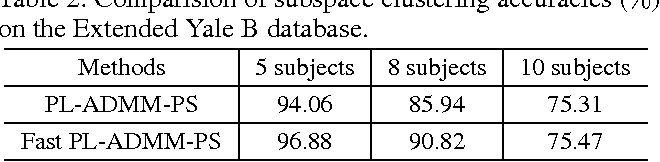The Augmented Lagragian Method (ALM) and Alternating Direction Method of Multiplier (ADMM) have been powerful optimization methods for general convex programming subject to linear constraint. We consider the convex problem whose objective consists of a smooth part and a nonsmooth but simple part. We propose the Fast Proximal Augmented Lagragian Method (Fast PALM) which achieves the convergence rate $O(1/K^2)$, compared with $O(1/K)$ by the traditional PALM. In order to further reduce the per-iteration complexity and handle the multi-blocks problem, we propose the Fast Proximal ADMM with Parallel Splitting (Fast PL-ADMM-PS) method. It also partially improves the rate related to the smooth part of the objective function. Experimental results on both synthesized and real world data demonstrate that our fast methods significantly improve the previous PALM and ADMM.

* AAAI 2016
Click to Read Paper